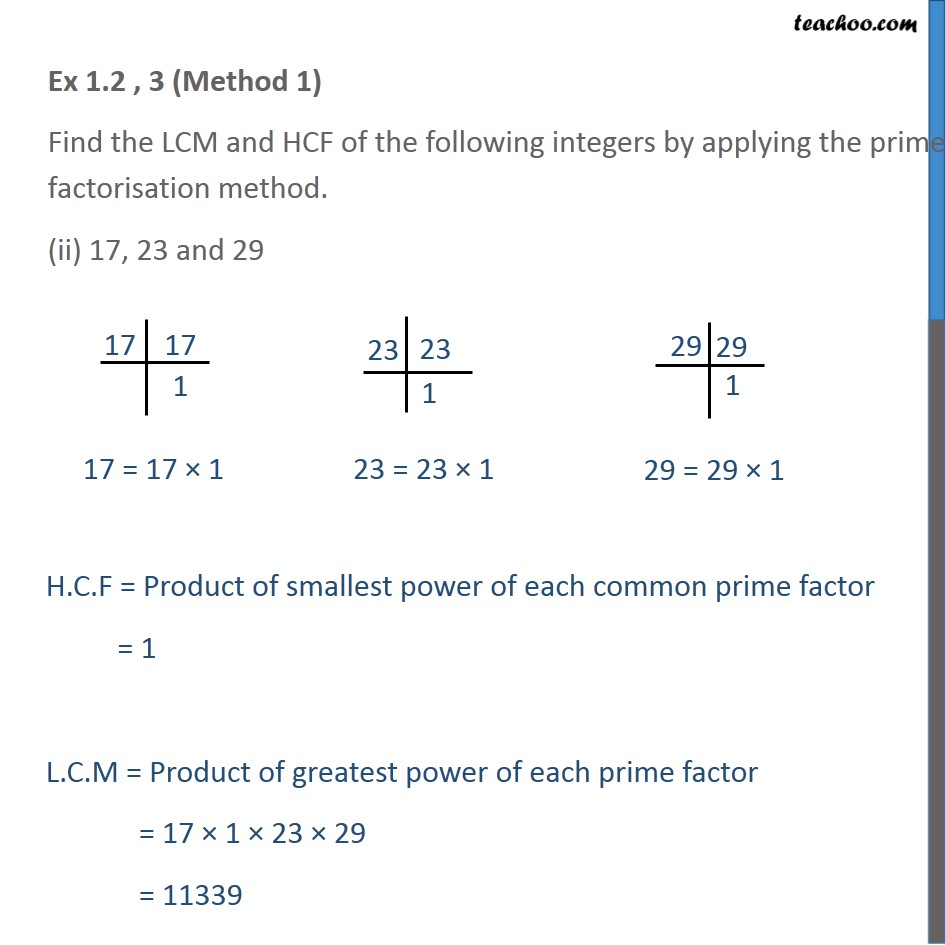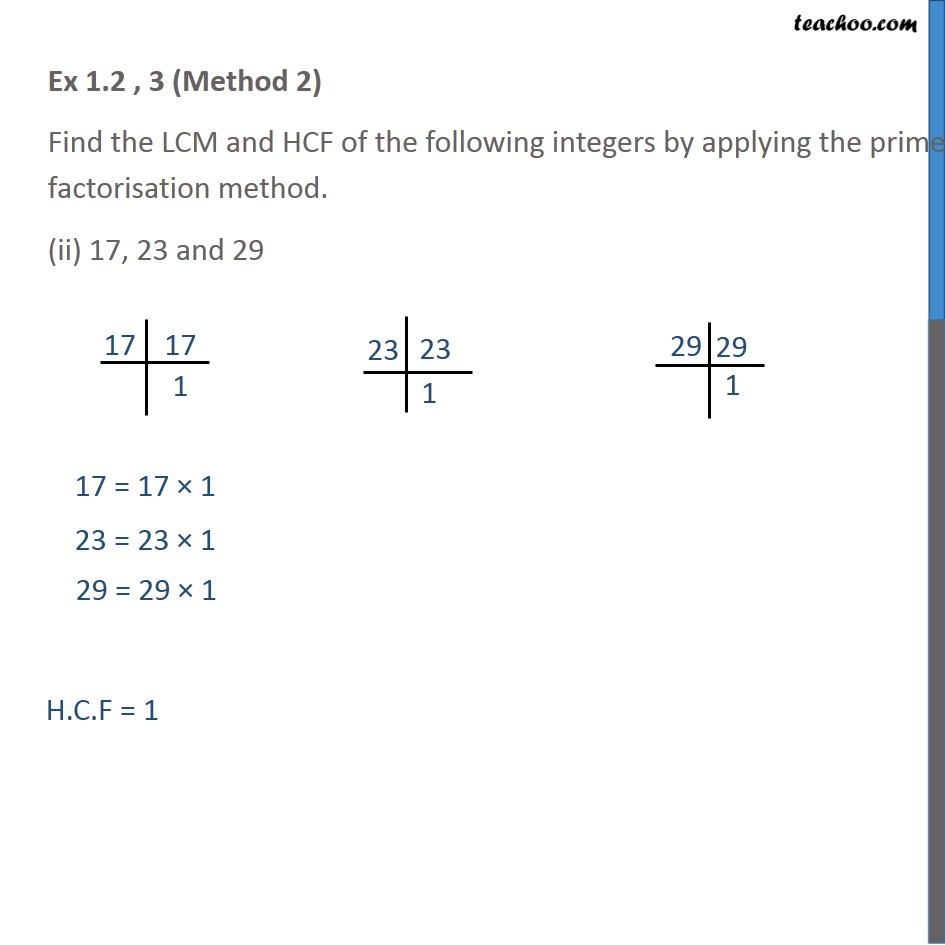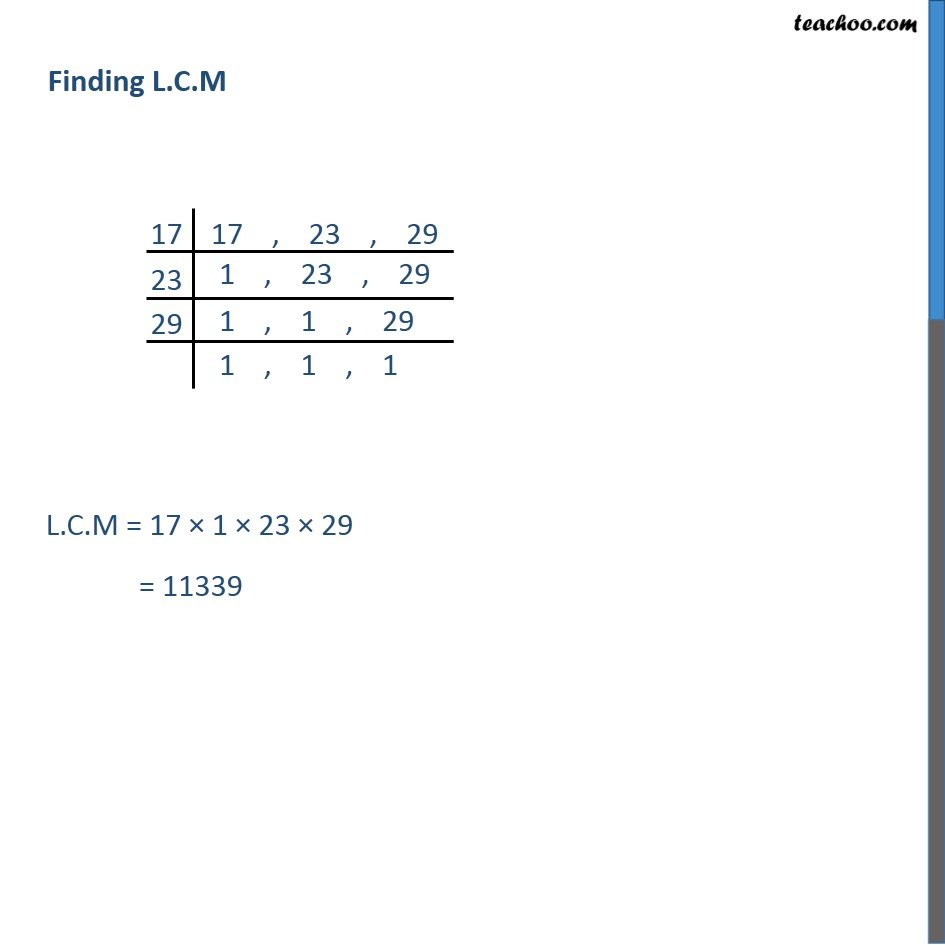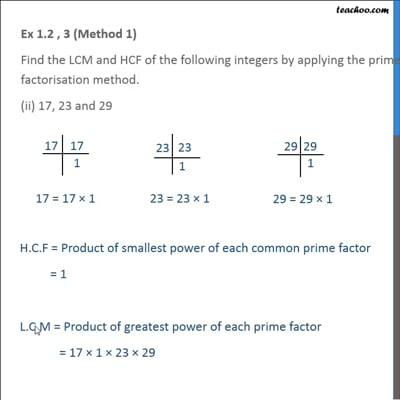Ex 1.2

Chapter 1 Class 10 Real Numbers
Serial order wiseThis video is only available for Teachoo black users

Introducing your new favourite teacher - Teachoo Black, at only ₹83 per month

### Transcript

Ex 1.2 , 3 (Method 1) Find the LCM and HCF of the following integers by applying the prime factorisation method. (ii) 17, 23 and 29 H.C.F = Product of smallest power of each common prime factor = 1 L.C.M = Product of greatest power of each prime factor = 17 × 1 × 23 × 29 = 11339 Ex 1.2 , 3 (Method 2) Find the LCM and HCF of the following integers by applying the prime factorisation method. (ii) 17, 23 and 29 H.C.F = 1 L.C.M = 17 × 1 × 23 × 29 = 11339